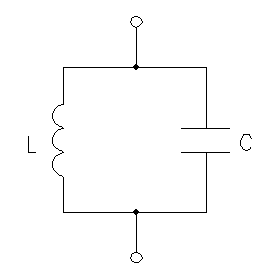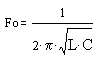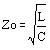# LC Tuned Circuit Calculator

Enter any two values of the first three (C, L, Fo) to calculate the third one and Characteristic Impedance Zo.Capacitance (C): pF nF µF mF F Inductance (L): pH nH µH mH H Frequency (Fo): Hz KHz MHz GHz THz Impedance (Zo): ohm Kohm Mohm